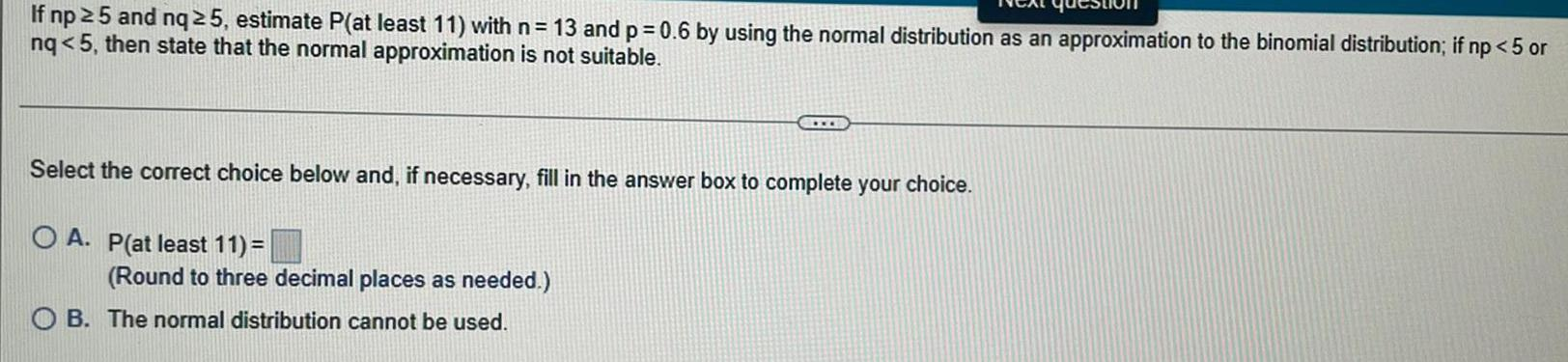Question:

# If np ≥ 5 and nq 25, estimate P(at least 11) with n = 13 and

Last updated: 7/7/2022If np ≥ 5 and nq 25, estimate P(at least 11) with n = 13 and p = 0.6 by using the normal distribution as an approximation to the binomial distribution; if np < 5 or nq<5, then state that the normal approximation is not suitable. Select the correct choice below and, if necessary, fill in the answer box to complete your choice. A. P(at least 11)= (Round to three decimal places as needed.) B. The normal distribution cannot be used.